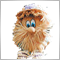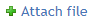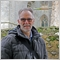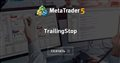# Can someone help me fix my EA trailing stop loss98

I coded a Simple Trail Stop EA from a tutorial video that i found on the net.

It compiled successfully with no errors. Here it is below.

```   #include  <Trade\Trade.mqh>

void OnTick()
{

//if we have no open positions
if (PositionsTotal()<1)

//open a test position 10 microlot ()dont do this on real account)

//Call the trailing stop module

}
//+------------------------------------------------------------------+
{

//check all open positions for current symbo
for(int i=PositionsTotal()-1; i>0; i--) //count all currency pair positions
{

string symbol=PositionGetSymbol(i); //Get position symbol

if (_Symbol==symbol) //if chart symbol equal position suymbol
{

//get the ticket number
ulong PositionTicket=PositionGetInteger(POSITION_TICKET);

{
//MODIFY the stop loss
}
}//if loop closed
}//end for loop
}// end breakeven stop function```

So i modified its parameters to use mine from the EA.

Now its giving me two errors;

'CheckBreakEvenStop' - undeclared identifier

I understand that i did not declare 'CheckBreakEvenStop' on that simple trail template on top and  'Ask' did not give me any problem.

But i am a bit confused why i have to declare this identifier and why an operator is missing on my EA code below.

In fact i am not sure where and how i have to declare  'CheckBreakEvenStop', neither for 'Ask'

So i need your help to get this Trailing Stop working as soon as EA opens a position.

For 'Bid' i will use your solution

```#property copyright "Copyright 2020, JayBee"
#property version   "1.00"

//---
CPositionInfo  m_position;                   // object of CPositionInfo class
CSymbolInfo    m_symbol;                     // object of CSymbolInfo class
CAccountInfo   m_account;                    // object of CAccountInfo class
//--- input parameters
input group             "RSILevels"
input int                  InpLower             = 85;
input int                  InpUpper             = 15;
input group             "MA"
input int                  Inp_MA_ma_period     = 7;           // MA Slow: averaging period
input int                  Inp_MA_ma_shift      = 0;           // MA Slow: horizontal shift
input ENUM_MA_METHOD       Inp_MA_ma_method     = MODE_SMA;    // MA Slow: smoothing type
input ENUM_APPLIED_PRICE   Inp_MA_applied_price = PRICE_CLOSE; // MA Slow: type of price
input group             "RSI"
input int                RSIPeriod       =  6;                // period of RSI
input ENUM_APPLIED_PRICE applied_price   =  PRICE_CLOSE;      // type of price
input int      InpTakeProfitPts     =  100;         //Take profit points
input int      InpStopLossPts       =  10000;       //Stop loss points
input int      InpTrailingStop      =  5000;        //Trail Stop loss points
input int      InpTrailingStep      =  50;          //Trail Step loss points
input double   InpOrderSize         =  0.20;        //Order size
input bool     InpPrintLog          =  false;       // Print log
input ulong    InpDeviation         =  10;          // Deviation
input ulong    InpMagic             =  931302198;   // Magic number
//---
bool     m_need_close_sells         = false;    // close all SELL positions
bool     m_need_open_sell           = false;    // open SELL position
datetime m_prev_bars                = 0;        // "0" -> D'1970.01.01 00:00';
//------declare variables
int      HandleMA;       // variable for storing the handle of the iMA indicator
int      HandleRSI;      // variable for storing the handle of the iRSI indicator
int      HandleMAonRSI;  // Handle of 7 Moving average of RSI
bool     Trigger;        //signal
//+------------------------------------------------------------------+
//| Expert initialization function                                   |
//+------------------------------------------------------------------+
int OnInit()
{
//---
ResetLastError();
if(!m_symbol.Name(Symbol())) // sets symbol name
{
Print(__FILE__," ",__FUNCTION__,", ERROR: CSymbolInfo.Name");
return(INIT_FAILED);
}
RefreshRates();
//---
//---create timer
EventSetTimer(60);

int   maPeriod  = 7;
int   rsiPeriod = 6;

//Calculations of indicators
//Calculating the handle for Moving average
HandleMA = iMA(Symbol(),Period(),maPeriod,0,MODE_SMA,PRICE_CLOSE);
//--- if the handle is not created
if (HandleMA==INVALID_HANDLE)
{
//--- tell about the failure and output the error code
PrintFormat("Failed to create handle of the iMA indicator for the symbol %s/%s, error code %d",
Symbol(),
EnumToString(Inp_MA_ma_method),
GetLastError());
//--- the indicator is stopped early
return(INIT_FAILED);
}
//Calculating the handle for RSI
HandleRSI = iRSI(Symbol(),Period(),rsiPeriod,PRICE_CLOSE);
//--- if the handle is not created
if (HandleRSI==INVALID_HANDLE)
{
//--- tell about the failure and output the error code
PrintFormat("Failed to create handle of the iRSI indicator for the symbol %s/%s, error code %d",
Symbol(),
EnumToString(applied_price),
GetLastError());
//--- the indicator is stopped early
return(INIT_FAILED);
}
//Calculating the handle for Moving average of the RSI
HandleMAonRSI = iMA(Symbol(),Period(),maPeriod,0,MODE_SMA,HandleRSI);
//--- if the handle is not created
if (HandleMAonRSI==INVALID_HANDLE)
{
//--- tell about the failure and output the error code
PrintFormat("Failed to create handle of the iMAOnRSI indicator for the symbol %s/%s, error code %d",
Symbol(),
EnumToString(Inp_MA_ma_method),
GetLastError());
//--- the indicator is stopped early
}
return(INIT_FAILED);
//---
return(INIT_SUCCEEDED);
}
//+------------------------------------------------------------------+
//| Expert deinitialization function                                 |
//+------------------------------------------------------------------+
void OnDeinit(const int reason)
{
//---destroy timer
EventKillTimer();

if (HandleMA!=INVALID_HANDLE) IndicatorRelease(HandleMA);
if (HandleRSI!=INVALID_HANDLE)IndicatorRelease(HandleRSI);
if (HandleMAonRSI!=INVALID_HANDLE)IndicatorRelease(HandleMAonRSI);

}
//+------------------------------------------------------------------+
//| Expert tick function                                             |
//+------------------------------------------------------------------+
void OnTick()
{

//We calculate the Bid price
double Bid=NormalizeDouble(SymbolInfoDouble(_Symbol,SYMBOL_BID),_Digits);

//+------------------------------------------------------------------+
//--- Condition for closing BUY positions OR SELL positions          |
//+------------------------------------------------------------------+

{
int count_sells   = 0;
//---
{
{
return;
}
else
}
//---
if(m_need_close_sells)
{
if(count_sells>0)
{
ClosePositions(POSITION_TYPE_SELL);
return;
}
else
m_need_close_sells=false;
}
}

//+------------------------------------------------------------------+
//+------------------------------------------------------------------+

{
if(!RefreshRates())
return;
//--- check volume before OrderSend to avoid "not enough money" error (CTrade)
double free_margin_check=m_account.FreeMarginCheck(m_symbol.Name(),
m_symbol.LotsMin(),
double margin_check=m_account.MarginCheck(m_symbol.Name(),
m_symbol.LotsMin(),
if(free_margin_check>margin_check)
{
if(InpPrintLog)

//if we have no positions open
if (PositionsTotal()<1)

//Call the trailing stop module

}
}
//+------------------------------------------------------------------+
//--- OPEN SELL POSITION                                             |
//+------------------------------------------------------------------+

if(m_need_open_sell)
{
if(!RefreshRates())
return;
//--- check volume before OrderSend to avoid "not enough money" error (CTrade)
double free_margin_check=m_account.FreeMarginCheck(m_symbol.Name(),
ORDER_TYPE_SELL,
m_symbol.LotsMin(),
double margin_check=m_account.MarginCheck(m_symbol.Name(),
ORDER_TYPE_SELL,
m_symbol.LotsMin(),
if(free_margin_check>margin_check)
{
if(InpPrintLog)
Print(__FILE__," ",__FUNCTION__,", OK: ","Signal SELL");
}
m_need_open_sell=false;
}
//--- we work only at the time of the birth of new bar
datetime time_0=iTime(m_symbol.Name(),Period(),0);
if(time_0==m_prev_bars)
return;
m_prev_bars=time_0;
//---
//---create buffers
double   maBuffer[];
double   rsiBuffer[];
double   MaonRsiBuffer[];
int      bufferNumber   = 0;
int      cnt;
int      required       = 2;
int      start          = 1;

cnt = CopyBuffer(HandleMA,bufferNumber,start,required,maBuffer);
if (cnt<required) return;
cnt = CopyBuffer(HandleRSI,bufferNumber,start,required,rsiBuffer);
if (cnt<required) return;
cnt = CopyBuffer(HandleMAonRSI,bufferNumber,start,required,MaonRsiBuffer);
if (cnt<required) return;

ArraySetAsSeries(MaonRsiBuffer,true);
int start_pos=0,count=6;
if(!iGetArray(HandleMAonRSI,0,start_pos,count,MaonRsiBuffer))
{
m_prev_bars=0;
return;
}
//--- check signal close SELL and open BUY
if (MaonRsiBuffer<=12 )
if (MaonRsiBuffer>10 )
{
m_need_close_sells=true;
return;
}
//--- check signal close BUY and open SELL
if (MaonRsiBuffer>=75 )
if (MaonRsiBuffer<77 )
{
m_need_open_sell=true;
return;
}
}
//+------------------------------------------------------------------+
//| Refreshes the symbol quotes data                                 |
//+------------------------------------------------------------------+
bool RefreshRates()
{
//--- refresh rates
if(!m_symbol.RefreshRates())
{
if(InpPrintLog)
Print(__FILE__," ",__FUNCTION__,", ERROR: ","RefreshRates error");
return(false);
}
//--- protection against the return value of "zero"
{
if(InpPrintLog)
Print(__FILE__," ",__FUNCTION__,", ERROR: ","Ask == 0.0 OR Bid == 0.0");
return(false);
}
//---
return(true);
}
//+------------------------------------------------------------------+
//| Get value of buffers                                             |
//+------------------------------------------------------------------+
bool iGetArray(const int handle,const int buffer,const int start_pos,
const int count,double &arr_buffer[])
{
bool result=true;
if(!ArrayIsDynamic(arr_buffer))
{
if(InpPrintLog)
PrintFormat("ERROR! EA: %s, FUNCTION: %s, this a no dynamic array!",__FILE__,__FUNCTION__);
return(false);
}
ArrayFree(arr_buffer);
//--- reset error code
ResetLastError();
//--- fill a part of the iBands array with values from the indicator buffer
int copied=CopyBuffer(handle,buffer,start_pos,count,arr_buffer);
if(copied!=count)
{
//--- if the copying fails, tell the error code
if(InpPrintLog)
PrintFormat("ERROR! EA: %s, FUNCTION: %s, amount to copy: %d, copied: %d, error code %d",
__FILE__,__FUNCTION__,count,copied,GetLastError());
//--- quit with zero result - it means that the indicator is considered as not calculated
return(false);
}
return(result);
}
//+------------------------------------------------------------------+
//| Calculate all positions                                          |
//+------------------------------------------------------------------+
{
count_sells = 0;
for(int i=PositionsTotal()-1; i>=0; i--)
if(m_position.SelectByIndex(i)) // selects the position by index for further access to its properties
if(m_position.Symbol()==m_symbol.Name() && m_position.Magic()==InpMagic)
{
else
if(m_position.PositionType()==POSITION_TYPE_SELL)
count_sells++;
}
}

//+------------------------------------------------------------------+
//| Close positions                                                  |
//+------------------------------------------------------------------+
void ClosePositions(const ENUM_POSITION_TYPE pos_type)
{
for(int i=PositionsTotal()-1; i>=0; i--) // returns the number of current positions
if(m_position.SelectByIndex(i)) // selects the position by index for further access to its properties
if(m_position.Symbol()==m_symbol.Name() && m_position.Magic()==InpMagic)
if(m_position.PositionType()==pos_type)
if(!m_trade.PositionClose(m_position.Ticket())) // close a position by the specified m_symbol
if(InpPrintLog)
}
//+------------------------------------------------------------------+

{
//set desired stoploss to 10000points

//check all open positions for the current symbol
for(int i=PositionsTotal()-1; i>=0; i--) //count all currency pair positions

{
string symbol = PositionGetSymbol(i); //get position symbol

if (_Symbol== symbol) //if chart symbol equals position symbol
{
//get the ticket number
ulong PositionTicket = PositionGetInteger(POSITION_TICKET);

//get the current stop loss
double CurrentStopLoss= PositionGetDouble(POSITION_SL);

//if current stop loss is below 150 points from ask price
if (CurrentStopLoss<SL)

{
//modify the stoploss by 5000 points
}

}//end symbol if loop

}  //end for loop

} //end trailing stop function```Moderator
233188

I always use Styler:

```//+------------------------------------------------------------------+
//|                                                      ProjectName |
//|                                       http://www.companyname.net |
//+------------------------------------------------------------------+
//--- create an instance of CTrade
//+------------------------------------------------------------------+
//| Expert tick function                                             |
//+------------------------------------------------------------------+
void OnTick()
{
//--- we calculate the Ask price
//--- if we have no open positions
if(PositionsTotal()<1)
//open a test position 10 microlot ()dont do this on real account)
//--- call the trailing stop module
}
//+------------------------------------------------------------------+
//| CheckBreakEvenStop                                               |
//+------------------------------------------------------------------+
{
//--- check all open positions for current symbo
for(int i=PositionsTotal()-1; i>0; i--) //count all currency pair positions
{
string symbol=PositionGetSymbol(i); //Get position symbol
if(_Symbol==symbol)  //if chart symbol equal position suymbol
{
//--- get the ticket number
ulong PositionTicket=PositionGetInteger(POSITION_TICKET);
//--- get the position buy price
{
//--- MODIFY the stop loss
}
}// if loop closed
}// end for loop
}// end breakeven stop function
//+------------------------------------------------------------------+```

This code is much easier to read.

Please do not forget to attach the code as a fileFiles:
1.mq5 5 kb98

Great...

1. i used your Styler and modified the figure for trailing stop from 200 to 1000 points.

2. since i need 2 Trailing stops, 1 for Buy and 1 for Sell, i renamed ''CheckBreakEvenStop''

For Sell positions i renamed to  ''CheckSellBreakEvenStop''

3. i called the trailing stop module for buy positions on line 221 like this

```//--- check signal close SELL and open BUY
if (MaonRsiBuffer<=30 )
if (MaonRsiBuffer>29 )
{
m_need_close_sells=true;
//--- call the trailing stop module
return;
}```

then called the trailing stop module for sell positions on line 231

```//--- check signal close BUY and open SELL
if (MaonRsiBuffer>=75 )
if (MaonRsiBuffer<77 )
{
m_need_open_sell=true;
//--- call the trailing stop module
CheckSellBreakEvenStop(Bid);
return;
}```

the code compiled with no errors but the Trail stop is not active i am not sure why. could you check for me why its not trailing.

Here is the full code

```//+------------------------------------------------------------------+
//|                                  MARSI EA stoploss n trailSL.mq5 |
//|                                     Copyright 2020, Brian M Jaka |
//|                                             https://www.mql5.com |
//+------------------------------------------------------------------+
#property version   "1.00"

//---
CPositionInfo  m_position;                   // object of CPositionInfo class
CSymbolInfo    m_symbol;                     // object of CSymbolInfo class
CAccountInfo   m_account;                    // object of CAccountInfo class
//--- input parameters
input group             "RSILevels"
input int                  InpLower             = 85;
input int                  InpUpper             = 15;
input group             "MA"
input int                  Inp_MA_ma_period     = 7;           // MA Slow: averaging period
input int                  Inp_MA_ma_shift      = 0;           // MA Slow: horizontal shift
input ENUM_MA_METHOD       Inp_MA_ma_method     = MODE_SMA;    // MA Slow: smoothing type
input ENUM_APPLIED_PRICE   Inp_MA_applied_price = PRICE_CLOSE; // MA Slow: type of price
input group             "RSI"
input int                RSIPeriod       =  6;                // period of RSI
input ENUM_APPLIED_PRICE applied_price   =  PRICE_CLOSE;      // type of price
input int      InpTakeProfitPts     =  100;         //Take profit points
input int      InpStopLossPts       =  10000;       //Stop loss points
input double   InpOrderSize         =  0.20;        //Order size
input bool     InpPrintLog          =  false;       // Print log
input ulong    InpDeviation         =  10;          // Deviation
input ulong    InpMagic             =  931302198;   // Magic number
//---
bool     m_need_close_sells         = false;    // close all SELL positions
bool     m_need_open_sell           = false;    // open SELL position
datetime m_prev_bars                = 0;        // "0" -> D'1970.01.01 00:00';
//------declare variables
int      HandleMA;       // variable for storing the handle of the iMA indicator
int      HandleRSI;      // variable for storing the handle of the iRSI indicator
int      HandleMAonRSI;  // Handle of 7 Moving average of RSI
bool     Trigger;        //signal

//+------------------------------------------------------------------+
//| Expert initialization function                                   |
//+------------------------------------------------------------------+
int OnInit()
{
//---
ResetLastError();
if(!m_symbol.Name(Symbol())) // sets symbol name
{
Print(__FILE__," ",__FUNCTION__,", ERROR: CSymbolInfo.Name");
return(INIT_FAILED);
}
RefreshRates();
//---
//---create timer
EventSetTimer(60);

int   maPeriod  = 7;
int   rsiPeriod = 6;

//Calculations of indicators
//Calculating the handle for Moving average
HandleMA          = iMA(Symbol(),Period(),
maPeriod,0,MODE_SMA,PRICE_CLOSE);
if (HandleMA==INVALID_HANDLE) return(INIT_FAILED);
//Calculating the handle for RSI
HandleRSI         = iRSI(Symbol(),Period(),
rsiPeriod,PRICE_CLOSE);
if (HandleRSI==INVALID_HANDLE) return(INIT_FAILED);
//Calculating the handle for Moving average of the RSI
HandleMAonRSI     = iMA(Symbol(),Period(),
maPeriod,0,MODE_SMA,HandleRSI);
if (HandleMAonRSI==INVALID_HANDLE) return(INIT_FAILED);
//---
return(INIT_SUCCEEDED);
}
//+------------------------------------------------------------------+
//| Expert deinitialization function                                 |
//+------------------------------------------------------------------+
void OnDeinit(const int reason)
{
//---destroy timer
EventKillTimer();
if (HandleMA!=INVALID_HANDLE) IndicatorRelease(HandleMA);
if (HandleRSI!=INVALID_HANDLE)IndicatorRelease(HandleRSI);
if (HandleMAonRSI!=INVALID_HANDLE)IndicatorRelease(HandleMAonRSI);
}
//+------------------------------------------------------------------+
//| Expert tick function                                             |
//+------------------------------------------------------------------+
void OnTick()
{

//We calculate the Bid price
double Bid=NormalizeDouble(SymbolInfoDouble(_Symbol,SYMBOL_BID),_Digits);

//--- Condition for closing BUY positions OR SELL positions
{
int count_sells   = 0;
//---
{
{
return;
}
else
}
//---
if(m_need_close_sells)
{
if(count_sells>0)
{
ClosePositions(POSITION_TYPE_SELL);
return;
}
else
m_need_close_sells=false;
}
}
{
if(!RefreshRates())
return;
//--- check volume before OrderSend to avoid "not enough money" error (CTrade)
double free_margin_check=m_account.FreeMarginCheck(m_symbol.Name(),
m_symbol.LotsMin(),
double margin_check=m_account.MarginCheck(m_symbol.Name(),
m_symbol.LotsMin(),
if(free_margin_check>margin_check)
{
if(InpPrintLog)
}
}
//--- OPEN SELL POSITION
if(m_need_open_sell)
{
if(!RefreshRates())
return;
//--- check volume before OrderSend to avoid "not enough money" error (CTrade)
double free_margin_check=m_account.FreeMarginCheck(m_symbol.Name(),
ORDER_TYPE_SELL,
m_symbol.LotsMin(),
double margin_check=m_account.MarginCheck(m_symbol.Name(),
ORDER_TYPE_SELL,
m_symbol.LotsMin(),
if(free_margin_check>margin_check)
{
if(InpPrintLog)
Print(__FILE__," ",__FUNCTION__,", OK: ","Signal SELL");
}
m_need_open_sell=false;
}
//--- we work only at the time of the birth of new bar
datetime time_0=iTime(m_symbol.Name(),Period(),0);
if(time_0==m_prev_bars)
return;
m_prev_bars=time_0;
//---
//---create buffers
double   maBuffer[];
double   rsiBuffer[];
double   MaonRsiBuffer[];
int      bufferNumber   = 0;
int      cnt;
int      required       = 2;
int      start          = 1;

cnt = CopyBuffer(HandleMA,bufferNumber,start,required,maBuffer);
if (cnt<required) return;
cnt = CopyBuffer(HandleRSI,bufferNumber,start,required,rsiBuffer);
if (cnt<required) return;
cnt = CopyBuffer(HandleMAonRSI,bufferNumber,start,required,MaonRsiBuffer);
if (cnt<required) return;

ArraySetAsSeries(MaonRsiBuffer,true);
int start_pos=0,count=6;
if(!iGetArray(HandleMAonRSI,0,start_pos,count,MaonRsiBuffer))
{
m_prev_bars=0;
return;
}
//--- check signal close SELL and open BUY
if (MaonRsiBuffer<=30 )
if (MaonRsiBuffer>29 )
{
m_need_close_sells=true;
//--- call the trailing stop module
return;
}
//--- check signal close BUY and open SELL
if (MaonRsiBuffer>=75 )
if (MaonRsiBuffer<77 )
{
m_need_open_sell=true;
//--- call the trailing stop module
CheckSellBreakEvenStop(Bid);
return;
}
}
//+------------------------------------------------------------------+
//| Refreshes the symbol quotes data                                 |
//+------------------------------------------------------------------+
bool RefreshRates()
{
//--- refresh rates
if(!m_symbol.RefreshRates())
{
if(InpPrintLog)
Print(__FILE__," ",__FUNCTION__,", ERROR: ","RefreshRates error");
return(false);
}
//--- protection against the return value of "zero"
{
if(InpPrintLog)
Print(__FILE__," ",__FUNCTION__,", ERROR: ","Ask == 0.0 OR Bid == 0.0");
return(false);
}
//---
return(true);
}
//+------------------------------------------------------------------+
//| Get value of buffers                                             |
//+------------------------------------------------------------------+
bool iGetArray(const int handle,const int buffer,const int start_pos,
const int count,double &arr_buffer[])
{
bool result=true;
if(!ArrayIsDynamic(arr_buffer))
{
if(InpPrintLog)
PrintFormat("ERROR! EA: %s, FUNCTION: %s, this a no dynamic array!",__FILE__,__FUNCTION__);
return(false);
}
ArrayFree(arr_buffer);
//--- reset error code
ResetLastError();
//--- fill a part of the iBands array with values from the indicator buffer
int copied=CopyBuffer(handle,buffer,start_pos,count,arr_buffer);
if(copied!=count)
{
//--- if the copying fails, tell the error code
if(InpPrintLog)
PrintFormat("ERROR! EA: %s, FUNCTION: %s, amount to copy: %d, copied: %d, error code %d",
__FILE__,__FUNCTION__,count,copied,GetLastError());
//--- quit with zero result - it means that the indicator is considered as not calculated
return(false);
}
return(result);
}
//+------------------------------------------------------------------+
//| Calculate all positions                                          |
//+------------------------------------------------------------------+
{
count_sells = 0;
for(int i=PositionsTotal()-1; i>=0; i--)
if(m_position.SelectByIndex(i)) // selects the position by index for further access to its properties
if(m_position.Symbol()==m_symbol.Name() && m_position.Magic()==InpMagic)
{
else
if(m_position.PositionType()==POSITION_TYPE_SELL)
count_sells++;
}
}
//+------------------------------------------------------------------+
//| Close positions                                                  |
//+------------------------------------------------------------------+
void ClosePositions(const ENUM_POSITION_TYPE pos_type)
{
for(int i=PositionsTotal()-1; i>=0; i--) // returns the number of current positions
if(m_position.SelectByIndex(i)) // selects the position by index for further access to its properties
if(m_position.Symbol()==m_symbol.Name() && m_position.Magic()==InpMagic)
if(m_position.PositionType()==pos_type)
if(!m_trade.PositionClose(m_position.Ticket())) // close a position by the specified m_symbol
if(InpPrintLog)
}
//+------------------------------------------------------------------+
//+------------------------------------------------------------------+
{
//--- check all open positions for current symbo
for(int i=PositionsTotal()-1; i>0; i--) //count all currency pair positions
{
string symbol=PositionGetSymbol(i); //Get position symbol
if(_Symbol==symbol)  //if chart symbol equal position suymbol
{
//--- get the ticket number
ulong PositionTicket=PositionGetInteger(POSITION_TICKET);
//--- get the position buy price
{
//--- MODIFY the stop loss
}
}// if loop closed
}// end for loop
}// end breakeven stop function
//+------------------------------------------------------------------+
//| CheckBreakEvenStop for sell trades                                |
//+------------------------------------------------------------------+
void CheckSellBreakEvenStop(double Bid)
{
//--- check all open positions for current symbo
for(int i=PositionsTotal()-1; i>0; i--) //count all currency pair positions
{
string symbol=PositionGetSymbol(i); //Get position symbol
if(_Symbol==symbol)  //if chart symbol equal position suymbol
{
//--- get the ticket number
ulong PositionTicket=PositionGetInteger(POSITION_TICKET);
//--- get the position sell price
double PositionSellPrice=PositionGetDouble(POSITION_PRICE_OPEN);
//--- if current bid price is 1000 points below sell price
if(Bid < (PositionSellPrice - 1000* _Point))
{
//--- MODIFY the stop loss
}
}// if loop closed
}// end for loop
}// end breakeven stop function
//+------------------------------------------------------------------+

```Moderator
233188

Trailing should always be launched - for trailing it does not matter what signals from the indicator.98

Trailing should always be launched - for trailing it does not matter what signals from the indicator.

Its not trailing on that EA i have used strategy tester but no movement. Can you check for me what it is i am missing.Moderator
233188

JayB :

Its not trailing on that EA i have used strategy tester but no movement. Can you check for me what it is i am missing.

'PositionModify' is trailing. Trailing does not depend on any indicators. Trailing does not care what the indicators show there - the main task of trailing is to pull up Stop Loss to the price. Therefore, you need to throw this block away:

```//--- check signal close SELL and open BUY
if (MaonRsiBuffer<=30 )
if (MaonRsiBuffer>29 )
{
m_need_close_sells=true;
//--- call the trailing stop module
return;
}
//--- check signal close BUY and open SELL
if (MaonRsiBuffer>=75 )
if (MaonRsiBuffer<77 )
{
m_need_open_sell=true;
//--- call the trailing stop module
CheckSellBreakEvenStop(Bid);
return;
}```4434

Trailing should always be launched - for trailing it does not matter what signals from the indicator.

I changed the code as follow (as suggested). you still have to adjust the stops, I get the error message ... invalid stops ....

```//--- check signal close SELL and open BUY
if (MaonRsiBuffer<=30 )
if (MaonRsiBuffer>29 )
{
m_need_close_sells=true;
return;
}
//--- check signal close BUY and open SELL
if (MaonRsiBuffer>=75 )
if (MaonRsiBuffer<77 )
{
m_need_open_sell=true;
return;
}
//--- call the trailing stop module
//--- call the trailing stop module
CheckSellBreakEvenStop(Bid);
```Moderator
233188

LUC JACOBUS A VERHEECKE :

I changed the code as follow (as suggested). you still have to adjust the stops, I get the error message ... invalid stops ....

You did not specify: Do you get an error for trailing a BUY or SELL position?98

'PositionModify' is trailing. Trailing does not depend on any indicators. Trailing does not care what the indicators show there - the main task of trailing is to pull up Stop Loss to the price. Therefore, you need to throw this block away:

I removed those blocks.

I had set my Stop loss to 10000 points below entry for Buy and Trailing stop loss at 5000 points right.

The reason why i am saying its not trailing or working is because the trade is getting into profit for over 5000 points and Stop loss still remains at 10000 points below entry.

When price traces back, its passing through entry all the way until it hits the stop loss (10000pnts).

That means trail stop was supposed to move 5000 up when price from entry moved over 5000, thats what i expected.

Otherwise i would have made 5000 pnts loss in this case if Trail stop had worked.

Thats how i understand it. Maybe i am having a problem somewhereModerator
233188

How trailing works is shown in the pictures in the TrailingStop code .TrailingStop
• www.mql5.com
Пример советника с реализацией Trailing Stop.98

LUC JACOBUS A VERHEECKE:

I changed the code as follow (as suggested). you still have to adjust the stops, I get the error message ... invalid stops ....

i have also changed by putting this Check trailing stop function under the OnTick like this.

```void OnTick()
{

//We calculate the Bid price
double Bid=NormalizeDouble(SymbolInfoDouble(_Symbol,SYMBOL_BID),_Digits);

//--- call the trailing stop module
//--- call the trailing stop module
CheckSellBreakEvenStop(Bid);

```

It does not give me errors, but even if i remove the functions still no errors.

the trailing stop is not showing any movements either.

thats my worry

when price moves 5000 points in profit i expect to see the trail stop going up 5000 but its not going up on my strategy tester